Olympiad Test Level 1: Quantitative Aptitude & Reasoning- 2

# Olympiad Test Level 1: Quantitative Aptitude & Reasoning- 2

Test Description

## 10 Questions MCQ Test GK Olympiad for Class 7 | Olympiad Test Level 1: Quantitative Aptitude & Reasoning- 2

Olympiad Test Level 1: Quantitative Aptitude & Reasoning- 2 for Class 7 2022 is part of GK Olympiad for Class 7 preparation. The Olympiad Test Level 1: Quantitative Aptitude & Reasoning- 2 questions and answers have been prepared according to the Class 7 exam syllabus.The Olympiad Test Level 1: Quantitative Aptitude & Reasoning- 2 MCQs are made for Class 7 2022 Exam. Find important definitions, questions, notes, meanings, examples, exercises, MCQs and online tests for Olympiad Test Level 1: Quantitative Aptitude & Reasoning- 2 below.
Solutions of Olympiad Test Level 1: Quantitative Aptitude & Reasoning- 2 questions in English are available as part of our GK Olympiad for Class 7 for Class 7 & Olympiad Test Level 1: Quantitative Aptitude & Reasoning- 2 solutions in Hindi for GK Olympiad for Class 7 course. Download more important topics, notes, lectures and mock test series for Class 7 Exam by signing up for free. Attempt Olympiad Test Level 1: Quantitative Aptitude & Reasoning- 2 | 10 questions in 20 minutes | Mock test for Class 7 preparation | Free important questions MCQ to study GK Olympiad for Class 7 for Class 7 Exam | Download free PDF with solutions
 1 Crore+ students have signed up on EduRev. Have you?
Olympiad Test Level 1: Quantitative Aptitude & Reasoning- 2 - Question 1

### A playground is in the form of a rectangle having semicircles on the shorter sides. Find its area when the length of the rectangular portion is 80 m and the breadth is 42 m.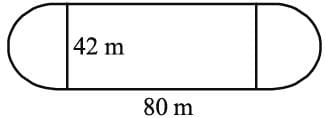Detailed Solution for Olympiad Test Level 1: Quantitative Aptitude & Reasoning- 2 - Question 1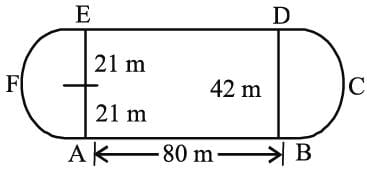Radius of each of semi-circle
= 42/2 = 21m
The required area =2 x area of semi-circle EFA + area of rectangle ABDE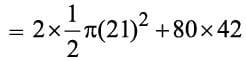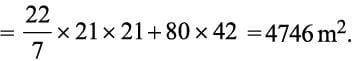Olympiad Test Level 1: Quantitative Aptitude & Reasoning- 2 - Question 2

### A playground which is 250 m long and 20 m broad is to be fenced with wire. How much wire is needed?

Detailed Solution for Olympiad Test Level 1: Quantitative Aptitude & Reasoning- 2 - Question 2

Clearly, the playground is of rectangular shape.
So the required length of the wire
= Perimeter of the rectangle
= 2 (length + breadth)
= 2 (250+20) = 540 m.

Olympiad Test Level 1: Quantitative Aptitude & Reasoning- 2 - Question 3

### Given a triangle ABC in which BC = 6 cm, ∠ABC = 120° and AB = 7 cm. What is the name of the triangle which can be drawn from the given data?

Detailed Solution for Olympiad Test Level 1: Quantitative Aptitude & Reasoning- 2 - Question 3

Obtuse angled triangle as ∠ABC = 120°.

Olympiad Test Level 1: Quantitative Aptitude & Reasoning- 2 - Question 4

Varun purchased a book and sold it at a profit of 20% to Vijay. If Vijay paid? ₹ 1440 to Varun, then at what price did Varun buy the book?

Detailed Solution for Olympiad Test Level 1: Quantitative Aptitude & Reasoning- 2 - Question 4

Let C.P. = ₹ x
C.P. + 20% of C.P. = S.P.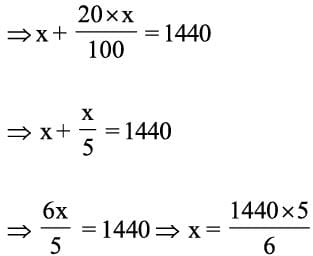= 240 x 5 = ₹ 1200

Olympiad Test Level 1: Quantitative Aptitude & Reasoning- 2 - Question 5

Three numbers A, B, and C are in the ratio 12 : 15 : 25. If the sum of these numbers is 312, then the ratio between the difference between B and A and the difference of C and B is

Detailed Solution for Olympiad Test Level 1: Quantitative Aptitude & Reasoning- 2 - Question 5

A : B : C = 12 : 15 :25
Total parts = 52
One part = 312/52 = 6
∴ A = 12 x 6 = 72, B = 15 x 6 = 90, C = 25 x 6 = 150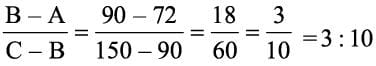Olympiad Test Level 1: Quantitative Aptitude & Reasoning- 2 - Question 6

The ratio of the age of a father to that of his son is 5 : 2. If the product of their ages in years is 1000, then find the father’s age after 10 years.

Detailed Solution for Olympiad Test Level 1: Quantitative Aptitude & Reasoning- 2 - Question 6

Let father’s age = x years and son’s age be y years
According to question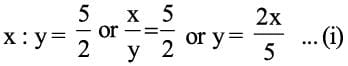Also; xy = 1000 .... (ii)
Put the value of y from Eqn. (i) in Eqn. (ii) we get
xy = 1000 ⇒ x × 2x/5 =1000 ⇒ 2x2 = 1000 × 5
⇒ x2 = 2500 ⇒ x = 50
So, after 10 years father’s age will be = x + 10
= 50+ 10 = 60 years.

Olympiad Test Level 1: Quantitative Aptitude & Reasoning- 2 - Question 7

If one angle of a triangle is equal to the sum of the other two angles, the triangle must be:

Detailed Solution for Olympiad Test Level 1: Quantitative Aptitude & Reasoning- 2 - Question 7

Let x, y and z is the angles, where x = y + z
x + y + z = 180° (angle sum property)
(y + z) + y + z = 180°
2(y + z)= 180°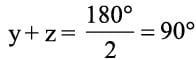x = 90°, hence triangle is right angled.

Olympiad Test Level 1: Quantitative Aptitude & Reasoning- 2 - Question 8

In the given figure, ∠a is greater than one-sixth of the right angle, then: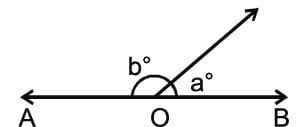Detailed Solution for Olympiad Test Level 1: Quantitative Aptitude & Reasoning- 2 - Question 8

As a > 1/6 (90°) => a > 15°
But a + b = 180° (linear Pair)
∴ b < 165°

Olympiad Test Level 1: Quantitative Aptitude & Reasoning- 2 - Question 9

If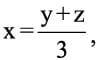find y in terms of x and z?

Detailed Solution for Olympiad Test Level 1: Quantitative Aptitude & Reasoning- 2 - Question 9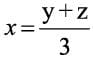⇒ 3x = y + z
⇒ 3 x - z = y

Olympiad Test Level 1: Quantitative Aptitude & Reasoning- 2 - Question 10

In an election between two candidates, one got 55% of the total valid votes, 20% of the votes were invalid. If the total number of votes was 7500, the number of valid votes that the other candidate got, was:

Detailed Solution for Olympiad Test Level 1: Quantitative Aptitude & Reasoning- 2 - Question 10

Total number of votes = 7500
Given that 20% of Percentage votes were invalid
⇒ Valid votes = 80%
Total valid votes = 7500*(80/100)
1st candidate got 55% of the total valid votes.
Hence the 2nd candidate should have got 45% of the total valid votes
⇒ Valid votes that 2nd candidate got = total valid votes x (45/100)
7500*(80/100)*(45/100) = 2700

## GK Olympiad for Class 7

63 tests
 Use Code STAYHOME200 and get INR 200 additional OFF Use Coupon Code
Information about Olympiad Test Level 1: Quantitative Aptitude & Reasoning- 2 Page
In this test you can find the Exam questions for Olympiad Test Level 1: Quantitative Aptitude & Reasoning- 2 solved & explained in the simplest way possible. Besides giving Questions and answers for Olympiad Test Level 1: Quantitative Aptitude & Reasoning- 2, EduRev gives you an ample number of Online tests for practice

63 tests